## ROUNDING

Rounding is mainly used in financial transactions which involve currency, payroll processing, invoicing, taxation, financial projections. many people do not use the rounding in Budgeting because this does not involves any financial  implication. personally i preferes to use this rounding when every money is involved.

Now we will see what are the rules of rounding as per Mathematics

Rules for Rounding off numbers.

In approximate computations it is frequently necessary to round off numbers (both approximate and exact), which means dropping one or more of the last digits. To ensure that the rounded number is as close as possible to the original number, use the following rules.

Rule 1. If the first of the discarded  digits exceeds 5, then the last digit kept is increased by unity. The increase is also made when the first digit kept is equal to 5 and is followed by one or more significant digits (for the case when the discarded 5 is not followed  by any digits, see Rule 3 below)

Rule 2. If the first digit dropped is less than 5, no increase is made

Rule 3. If the digit 5 is dropped, and no significant digits come after it, the rounding is done to the closest even number; that is the last retained digit is left unchanged if it is odd. The reason for this rule is given below.

Ex 1: Rounding 0.0465 to the third decimal place, we write 0.046. We do not increase the last digit kept since it is even. The number 0.046 is just as close to the given one as is 0.047

Ex 2: Rounding 0.935 to the second decimal place, we write 0.94. the last retained digit 3 is increased because it is odd.

Ex 3: Rounding the numbers, 6.527, 0.456, 2.195, 1.450, 0.950, 4.851, 0.850, 0.05 to the first decimal place, we get 6.5, 0.5, 2.2, 1.4, 1.0, 4.9, 0.8, 0.0

Note: When applying Rule 3 to the rounding off of one number do not increase the accuracy of the rounding process (Ex 1 and 2). However, if the process is performed repeatedly, there will be roughly just as many numbers with excess as with deficit. The mutual compensation of errors will ensure the highest possible accuracy  of the result.

Rule 3 can be modified and made to apply to rounding off to the closest odd number. The accuracy will be the same but even digits are more convenient than odd numbers.

Standard Rounding Rules:

1) select position you want to round to;

2) look 1 to the right and if that is 5 or greater, add 1; if it is 4 or less, don’t add 1; then remove all other digits.

The ROUND function follows the standard rounding rules and rounds to a specified digit

The ROUNDUP function always rounds up to a specified digit

The ROUNDDOWN function always rounds down to a specified digit

The MROUND function follows the standard rounding rules and rounds to a specified amount (multiple)

The CEILING function always rounds up to a specified amount (multiple)

The FLOOR function always rounds down to a specified amount (multiple)

Now lets see each function in details with their syntax and examples.

(1) The ROUND function returns a number rounded to the specified number of places.

The Syntax of the function is as follows:

ROUND(number, count)

number:  The number to be rounded. number is a number value.

count:  The number of digits you want to retain, relative to the decimal point. digits is a number value. A positive number represents digits (decimal places) to the right of the decimal point to include. A negative number specifies digits to the left of the decimal point to replace with zeros (the number of zeros at the end of the number).

All ways remember Decimal place ( . ) is significant in the round function. If the number argument is 2 then value spit out by the function will have upto two decimals. if the number is 0 then the only integer part will only will come. if the number argument is -2 then the number is rounded to nearest hundred this you can clearly see the following picture.

Example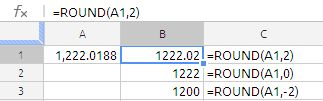(2) MROUND: The MROUND function rounds a number to the nearest multiple of a specified factor.

The Syntax of the function is as follows

MROUND(number, multiple)

number:  The number to be rounded. num-to-round is a number value.

multiple:  The number to use to determine the closet multiple. multiple is a number

value. It must have the same sign as number.

Examples:

MROUND(12.0188,5) returns 10

MROUND(12.7187,5) return 15

MROUND(14.99,5) return 15

MROUND(15.01,5) return 15

MROUND(17.6,5) return 20

(3)ROUNDUP :function returns a number rounded away from zero (rounded up) to the specified number of places.

The Syntax of the function is as follows:

ROUNDUP(number, count)

number:  The number to be rounded. number is a number value.

count:  The number of digits you want to retain, relative to the decimal point. digits is a number value. A positive number represents digits (decimal places) to the right of the decimal point to include. A negative number specifies digits to the left of the decimal point to replace with zeros (the number of zeros at the end of the number).

Example(4) ROUNDDOWN: The ROUNDDOWN function returns a number rounded toward zero (rounded down) to the specified number of places.

The Syntax of the functions is

ROUNDDOWN(number, count)

number:  The number to be rounded. number is a number value.

count:  The number of digits you want to retain, relative to the decimal point. digits is a number value. A positive number represents digits (decimal places) to the right of the decimal point to include. A negative number specifies digits to the left of the decimal point to replace with zeros (the number of zeros at the end of the number).

Example: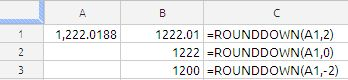(5) INT function: Some times INT is very useful to round the numbers

The INT function returns the nearest integer that is less than or equal to the number.

the syntax of the function is as follows:

INT(number)

number:  The number to be rounded. number is a number value.

Example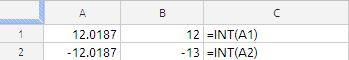(6) FLOOR :The FLOOR function rounds a number toward zero to the nearest multiple of the specified factor.

The Syntax of the function is as follows:

FLOOR(number, significance, mode)

number:  The number to be rounded. number is a number value.

significance:  The number to use to determine the closet multiple. factor is a number value. It must have the same sign as number.

mode: Mode is an optional value. If it is indicated and non-zero and if the number and significance are negative, rounding up is carried out based on that value.

CEILING: The CEILING function rounds a number away from zero to the nearest multiple of the specified factor.

The Syntax of the function is

CEILING(number, significance, mode)

number:  The number to be rounded. num-to-round is a number value.

significance:  The number to use to determine the closet multiple. multiple-factor is a number value and must have the same sign as num-to-round.

mode: Mode is an optional value. If it is indicated and non-zero and if the number and significance are negative, rounding up is carried out based on that value.

Example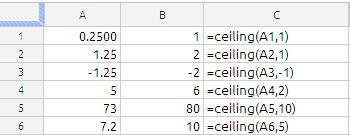TRUNC : The TRUNC function truncates a number to the specified number of digits.

Syntax of the function is as follows:

TRUNC(number, count)

number:  A number. number is a number value.

count:  An optional value specifying the number of digits you want to retain, relative to the decimal point. count is a number value. A positive number represents digits (decimal places) to the right of the decimal point to include. A negative number specifies digits to the left of the decimal point to replace with zeros (the number of zeros at the end of the number).

example: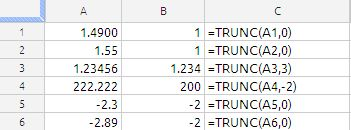Example of all functions combines on same data set.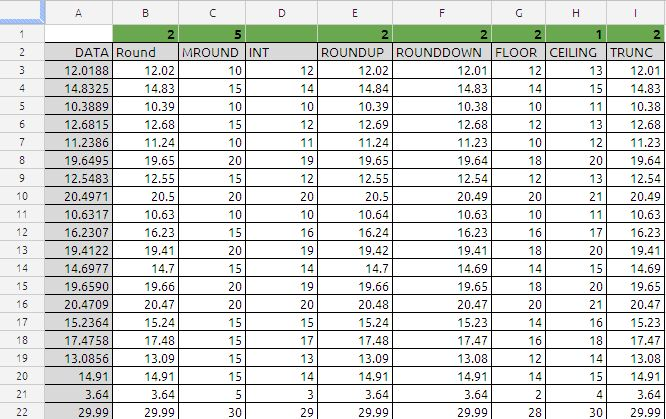In cell A2 to A22 are the data set and in cell B1 TO I1 are the second arguments of the each function except column D which INT which is single argument function.